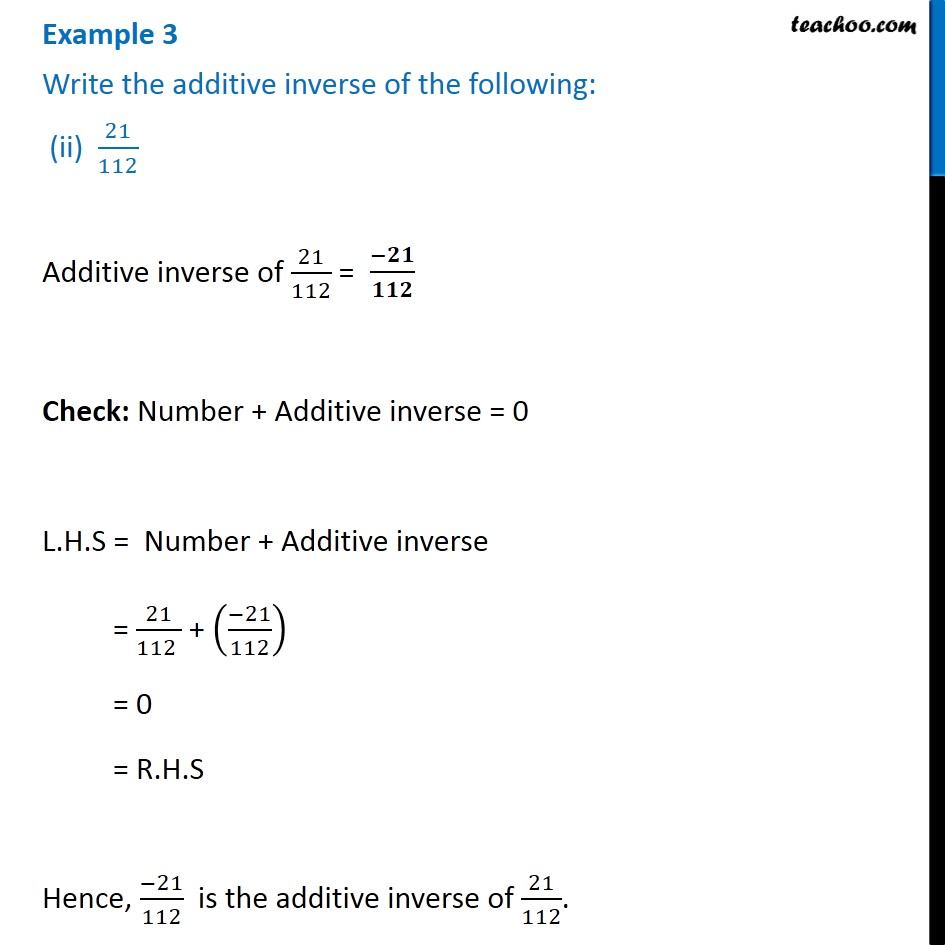Examples

Chapter 1 Class 8 Rational Numbers
Serial order wiseLearn in your speed, with individual attention - Teachoo Maths 1-on-1 Class

### Transcript

Example 3 Write the additive inverse of the following: (ii) 21/112 Additive inverse of 21/112 = Check: Number + Additive inverse = 0 L.H.S = Number + Additive inverse = 21/(112 ) + ((−21)/112) = 0 = R.H.S Hence, (−21)/112 is the additive inverse of 21/112.